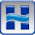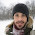## Tuesday, December 2, 2008

### Samuel's Equation for Cross Section Spacing

Written by Chris Goodell, P.E., D. WRE | WEST Consultants

I just got a call from a colleague of mine about Paul Samuel's equation for cross section spacing in 1-D unsteady flow models (like HEC-RAS). Samuel's equation reads DX = 0.15*D/S, where DX is the cross section spacing, D is the Depth and S is the bed slope. So the question was, which depth do you use?

Well Samuel's equation (Samuels, P.G., 1989. “Backwater lengths in rivers”, Proceedings -- Institution of Civil Engineers, Part 2, Research and Theory, 87, 571-582.) was developed for bankful depth. In his derivation, Samuel uses Manning's equation for conveyance, which is a function of the hydraulic radius. His switch to bankful depth for his final equation is two-fold. One for convenience (a depth characteristic is easier to measure in the field than hydraulic radius), but also because bankful depth typically yields the maximum hydraulic radius for typical flood flows in a natural channel.

That being said when running extreme events in HEC-RAS, such as a dam breach, more consideration for a larger depth should be given. Though conventional wisdom has been that closer cross sections for implicit solution schemes (like RAS) make for a more stable model, this is not necessarily true with HEC-RAS. And, in fact, cross section spacing too close can overestimate energy loss and precipitate significant error, and eventually numeric instability.

The bottom line, and I mention this every time I go over cross section spacing with students, is use an equation that seems to work for the rivers you work on to get a good starting point for spacing. Then adjust from there as necessary. You'll find that over time, you will rely more and more on the "eyeball" test, or gut-feel for determining spacing, rather than an equation or rule of thumb...

It's interesting to note that the first part of Samuel's paper derives a backwater length (thus the name of the paper). This is the length of channel affected by some disturbance downstream. The idea being that in the planning stage of a project, you could decide how long to make your model. This length (through a rather elegant numerical analysis of the St. Venant equations and Manning's equations, with a side order of Froude Number) turns out to be simply 0.7D/S. The 0.15 number in the cross section spacing equation is then simply (and very anti-climatically) 0.7 divided by 5, since in his experience, the number of cross sections needed to model a backwater reach is 5. For some reason, that is very funny to me...

1.Richard Taylor CFMJune 2, 2009 at 6:31 AM

Extremely useful information here. Using this equation for the project I am working on giving me a cross section interval of 250 feet. Based on my experience, I had already decided on an interval of 300 to 350 feet before working out the equation. It's nice to have some justification for the spacing of cross sections in our models.

2.hello.

thanks a lot for your USEFULL post. with the reference i just stopped by my university's library and found the original article from PG Samuels. i can scan the text for sharing in your blog if you want it. my email is axa_maqueda(at)hotmail.com

3.Hello.

Interesting blog, I learn a lot of new things.

I would like to know where I could find the paper about Samuel´s equation.

Thanks

4.Is 'feet' used as the unit of Depth in this equation?

1.Any unit of length will work. Feet, meters, etc. The equation is unitless.

2.5.You mention that "cross section spacing too close can overestimate energy loss and precipitate significant error" Do you have a sense of when these errors start to occur, i.e. what the sensitivity to spacing is and what threshold should we use to say we're too far off the equation based estimate?

1.Justin- Sorry to take so long to respond. This slipped by me. Anyway, to answer your question, not really. It seems to be different for every model. Most data sets have a "window" of workable spacings. In an ideal world, after I have a stable working model with good results, I'll test a range of spacings as well as timesteps to gage the sensitivity of the model to those parameters. In the real world of consulting, I usually don't have the budget to do that, so I start with Samuel's equation (and a little bit of "gut" feel) to get a first cut spacing of cross sections. Then I add more where needed until I have a nice stable, and reasonable-looking profile or profiles. Hope this helps. If you ever do get a chance to test the threshold of application about the equation's results, I'd love to see the results and get that up on the blog. Thanks-Chris

6.For many years I struggled with these seemingly arbitrary model selections…primarily how far to extend a model upstream and downstream of a structure of interest, and interval spacing of the cross-sections. A couple years ago I went to a workshop sponsored by the state’s DEQ and it was discussed that topo data should be collected near bends in the river and when there are changes to the bed slope. That is generally how I have subsequently been setting up my models since then. Having now just discovered this Samuel’s equation, I will consider using it for future cross-section spacing (to think…I’m on this site trying to research a solution to a different issue that I am having and in the meantime learning all kinds of cool things! :).

Anyhow, the other part of how far to extend a model…(this is typically in relation to bridge/culvert replacement studies)…I have decided, at least during the initial set up of the model, to ensure the model includes the start of contraction and end of expansion reaches and maybe an additional 50-100’ u/s and d/s of those set points. Now I have another tool to estimate an appropriate u/s distance. Thank you.

1.Great! You're welcome!

7.Hi Chris,

thanks a lot for your blog. I tried to run a 1D HEC-RAS project using three different configurations of cross-sections. The project concerns a 900 meters channel of rectangular shape.

The 1st configuration considers just two cross-sections (one at the beginning and one at the end of the channel I am working with).

The 2nd configuration takes into account three cross-sections (like the 1st configuration with a cross-section in the middle point of channel length).

The 3rd configuration has five cross-sections (equally distributed between the beginning and the end of the channel).

What I've noticed is the following: while the flow always remains within the channel banks in the first case (with likely flow depth between 40 cm at the beginning and 300 cm at the end of the channel), with the other two configurations I get overflow with meaningless depths (up to 150 m in the 2nd case between the 1st and the 2nd cross-section and up to about 400 m in the 3rd case along all the channel).

Have you any idea of these different behaviors among the three configurations? What I was expecting was some little differences among those.

Given that the only simulation having a sense is the first one, can I say it is the correct one? More generally, how can I set up the correct configuration of cross-sections to use?

Also, in the first case I get the following warning:
"The maximum xsec wsel error was 0.037 River 1 Reach 1 2869 at 24SEP2018 06:01:00". Di you know how can I fix it?